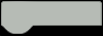# Xylophone Hero

Learn classic songs on a xylophone using LEDs as indicators for which note to hit, and when.HARDWARE LIST
1 Arduino (+ power cable)
1 LED strip (ALITOVE WS2811)
1 wires
1 220 ohm resistor
1 breadboard or blank circuit board
1 carboard to hold the LEDs in place

Story

I created this project as a way to learn some simple songs on the xylophone to play for my son.

Circuit:

-D7 -> resistor -> LED strip DIN

-GND -> LED strip GND

-+5v -> LED strip +5vArrange the LED strip in this pattern:The code is pretty simple, it just updates the strip every tick (depends on the pace of the song) and will skip to the next song after a song is done playing. Read the code here:

The interesting bits are:

How songs are encoded:

CODE
``````char *MARY_HAD_A_LITTLE_LAMB = "edcdeee_ddd_egg_edcdeeeeddedc___edcdeee_ddd_egg_edcdeeeeddedc___\0";
char *ITSY_BITSY_SPIDER = "_g_gg_AB__B_BA_gA_Bg_____B__B_CD__D__C_BC_DB_____g__g_AB__B__A_gA_Bg_____g_gg_AB__B_BA_gA_Bg__g__\0";
char *YOU_ARE_MY_SUNSHINE = "ccde_e__edec_c_cdef_A__Agfe___cdef_A__Agfe_c___cde__fd_dec___\0";
char *BINGO = "dggddeeddggAAB_g_B_B_CCC_A_A_BBB_/g_g_AAAgfdefg_g_\0";
char *SAINTS_MARCHING = "_cefg____cefg____cefg_e_c_e_d____eedc__ce_g_g_f__fefg_e_c_d_c___\0";
char *ROW_ROW_YOUR_BOAT = "c__c__c__de_e_de_fg__g__CCCgggeeecccg_fe_dc__c__c__c__c__de_e_de_fg__g__CCCgggeeecccg_fe_dc__c__\0";
...
// Get the index of a note
int getNoteIdx(char note) {
int noteInt = (int)note;
switch (note) {
case 'a': return 0; // = 97
case 'b': return 0;
case 'c': return 0;
case 'd': return 1;
case 'e': return 2;
case 'f': return 3;
case 'g': return 4; // = 103
case 'A': return 5; // = 65
case 'B': return 6;
case 'C': return 7;
case 'D': return 7;
case 'E': return 7;
case 'F': return 7;
case 'G': return 7; // = 71
default:
Serial.println("Broken note: " + String(note));
return -1;
}
}``````

Getting the LED index of a note (Remember the snake pattern the LEDs are in makes it more difficult):

CODE
``````// Get the index of the LED in the LED strip for the row and column
// trackIdx = the row (index within a single track)
// noteIdx = the column (index within the notes)
int getLEDIdx(int trackIdx, int noteIdx) {
// LED strips are alternating in direction, which is why there is 2 cases
return trackIdx % 2 == 0?
trackIdx * TRACKS + noteIdx : // Regular 2d lookup
trackIdx * TRACKS + (TRACKS - noteIdx - 1); // Regular 2d lookup with reversed column
}``````

And of course the main loop where LEDs are updated:

CODE
``````// Update the LEDs based on where we are in the song
// elapsed
void updateDisplayLEDs(long elapsed) {
// Calculate start and end index within notes
int startIdx = max(0, (elapsed - displayMillis) / beatTime);
int endIdx = elapsed / beatTime;
FastLED.clear();
for (int idx = startIdx; idx < strlen(notes) && idx <= endIdx; idx++) {
// Get the note using pointer arithmatic
char note = *(notes + idx);
if (note == '_') {
continue;
}
int noteIdx = getNoteIdx(note);
// Get the index within the note's track
float percentComplete = (float)(elapsed - (idx * beatTime)) / (float)displayMillis;
if (percentComplete >= 1.0) {
continue;
}
int trackIdx = min(TRACK_LENGTH - 1, floor(TRACK_LENGTH * percentComplete));
// Get the LED idx from track and noteIdx
int LEDIdx = getLEDIdx(trackIdx, noteIdx);
if (LEDIdx < 0 || LEDIdx >= NUM_LEDS) {
Serial.println("Out of bounds LEDIdx: " + String(LEDIdx) + " track: " + String(trackIdx) + " note: " + String(noteIdx));
}
// Color the LED
leds[LEDIdx] = colors[noteIdx];
}
FastLED.show();
} ``````

This was more of a software project than a hardware project.

The article was first published in hackster, May 22, 2022

cr: https://www.hackster.io/kschieck/xylophone-hero-cfd393

author: kschieck